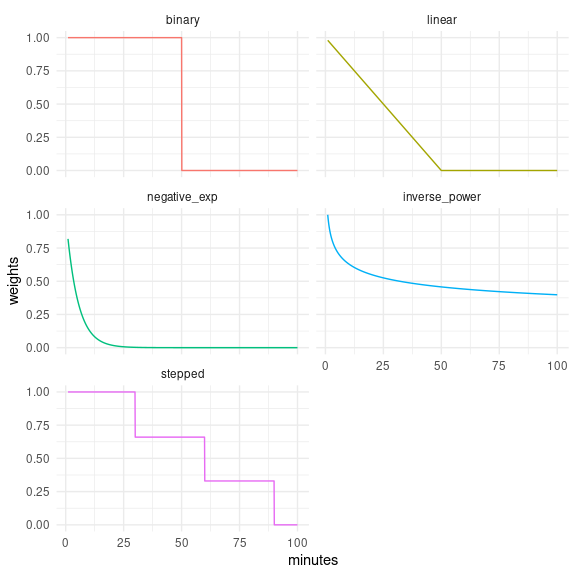# Decay functions

Some functions of the accessibility package, such as floating_catchment_area() and gravity(), use decay functions to continuously discount the weight of opportunities as travel costs become larger. For convenience, the package ships with some of the most frequently used functions in the accessibility literature, which are discussed below. Additionally, users can pass custom functions to convert travel costs into weights to be applied the opportunities, which is discussed further down the vignette.

# Currently available decay functions

## Binary

Also known as the step decay function, it’s most commonly used in cumulative opportunities measures.

\begin{aligned} f(t_{ij})= \left\{ \begin{array}{ll} 1 & \quad \text{for }t_{ij} \leq T \\ 0 & \quad \text{for }t_{ij} > T \end{array} \right.\\ \end{aligned}

Where $$t_{ij}$$ is the travel cost between origin i and destination j, and $$T$$ is the travel cost cutoff.

## Linear

Weights decay linearly until the travel cost cutoff is reached. From this point onward weights assume the value of 0.

\begin{aligned} f(t_{ij})= \left\{ \begin{array}{ll} (1 - t_{ij}/ T) & \quad \text{for }t_{ij} \leq T \\ 0 & \quad \text{for }t_{ij} > T \end{array} \right.\\ \end{aligned}

Where $$t_{ij}$$ is the travel cost between origin i and destination j, and $$T$$ is the travel cost cutoff.

## Negative exponential

\begin{aligned} f(t_{ij})= e^{(-\beta t_{ij})} \end{aligned}

Where $$t_{ij}$$ is the travel cost between origin i and destination j, and $$\beta$$ is the parameter that tells the speed of decay.

## Inverse power

\begin{aligned} f(t_{ij})= \left\{ \begin{array}{ll} 1 & \quad \text{for } t_{ij}\leq 1 \\ t_{ij}^{-\beta} & \quad \text{for }t_{ij} > 1 \end{array} \right.\\ \end{aligned}

Where $$t_{ij}$$ is the travel cost between origin i and destination j, and $$\beta$$ is the parameter that tells the speed of decay.

## Stepped

Similar to the binary function, but can take an arbitrary number of steps. The current implementation assumes that values changes at each step, instead of right after it.

\begin{aligned} f(t_{ij})= \left\{ \begin{array}{ll} 1 & \quad \text{for } t_{ij} \lt S_{1} \\ v_{1} & \quad \text{for } t_{ij} \lt S_{2} \\ v_{2} & \quad \text{for } t_{ij} \lt S_{3} \\ ... \\ v_{n-1} & \quad \text{for } t_{ij} \lt S_{n} \\ v_{n} & \quad \text{otherwise} \\ \end{array} \right.\\ \end{aligned}

Where $$t_{ij}$$ is the travel cost between origin i and destination j, $$n$$ is the total number of steps, $$S_{k}$$ is the travel cost cutoff that delimits the $$k^{th}$$ step, and $$v_{k}$$ is the value that the decay function assumes at the $$k^{th}$$ step.

### Quick demonstration

library(accessibility)
library(data.table)
library(ggplot2)

binary <- decay_binary(cutoff = 50)
linear <- decay_linear(cutoff = 50)
negative_exp <- decay_exponential(decay_value = 0.2)
inverse_power <- decay_power(decay_value = 0.2)
stepped <- decay_stepped(steps = c(30, 60, 90), weights = c(0.66, 0.33, 0))

travel_costs <- seq(1, 100, 0.1)

weights <- data.table(
minutes = travel_costs,
binary = as.numeric(binary(travel_costs)),
linear = linear(travel_costs),
negative_exp = negative_exp(travel_costs),
inverse_power = inverse_power(travel_costs),
stepped = stepped(travel_costs)
)

# reshape data to long format
weights <- melt(
weights,
id.vars = "minutes",
variable.name = "decay_function",
value.name = "weights"
)

ggplot(weights) +
geom_line(
aes(minutes, weights, color = decay_function),
show.legend = FALSE
) +
facet_wrap(. ~ decay_function, ncol = 2) +
theme_minimal()# Using a custom decay function

Any function that takes a numeric vector of travel costs and returns a numeric vector, with the same length of input, of weights to be applied to the opportunities can be considered a decay function.

Let’s say that we wanted to use a very simple decay function that defines the weights as the multiplicative inverse of travel cost - i.e. $$travel\_cost^{-1}$$. We just have to take care of the case when travel cost is less than 1, in which case the function would return values greater than 1 and which we will replace with 1, but otherwise the implementation is pretty simple:

my_decay <- function(travel_cost) {
weights <- 1 / travel_cost
weights[weights > 1] <- 1
return(weights)
}

Given a numeric vector of travel costs, the function returns a numeric vector of weights:

my_decay(c(0, 0.5, 1, 2, 5, 10))
#>  1.0 1.0 1.0 0.5 0.2 0.1

Using this function to calculate accessibility is as easy as any of the built-in decay functions:

data_dir <- system.file("extdata", package = "accessibility")

travel_matrix <- readRDS(file.path(data_dir, "travel_matrix.rds"))
land_use_data <- readRDS(file.path(data_dir, "land_use_data.rds"))

custom_gravity <- gravity(
travel_matrix,
land_use_data,
opportunity = "jobs",
travel_cost = "travel_time",
decay_function = my_decay
)
#>                 id     jobs
#> 1: 89a88cdb57bffff 11210.42
#> 2: 89a88cdb597ffff 10775.77
#> 3: 89a88cdb5b3ffff 11480.25
#> 4: 89a88cdb5cfffff 12689.44
#> 5: 89a88cd909bffff 11361.66
#> 6: 89a88cd90b7ffff 12563.65

In fact, we could achieve the exact same result as we did with our custom function using decay_power(1):

power_gravity <- gravity(
travel_matrix,
land_use_data,
opportunity = "jobs",
travel_cost = "travel_time",
decay_function = decay_power(1)
)
#>                 id     jobs
#> 1: 89a88cdb57bffff 11210.42
#> 2: 89a88cdb597ffff 10775.77
#> 3: 89a88cdb5b3ffff 11480.25
#> 4: 89a88cdb5cfffff 12689.44
#> 5: 89a88cd909bffff 11361.66
#> 6: 89a88cd90b7ffff 12563.65

A small difference is that in the first example we passed my_decay (the function object) to the decay_function parameter, whereas in the second we passed the function call decay_power(1). That’s because decay_power() (and the other built-in decay functions as well) is actually a function “factory”: it’s a function that returns a function, as we can see below:

decay_power(1)
#> function (travel_cost)
#> {
#>     weights <- travel_cost^(-decay_value)
#>     weights[weights > 1] <- 1
#>     return(weights)
#> }
#> <bytecode: 0x557b08b4cec0>
#> <environment: 0x557b0849b958>

This is because the built-in functions aim to be as generally used as possible, so we have to able to use them with different parameters, cutoffs, etc. In practice, every time we call these function we create another function that calculates travel costs exactly as we want.

When we create our own custom decay function, we don’t have to think about general cases, just the cases that matter for us. Creating a simple function that converts one vector into another, therefore, suffices our needs. In both examples above, therefore, we’re passing a function to decay_function - one we created ourselves and another created by decay_power().